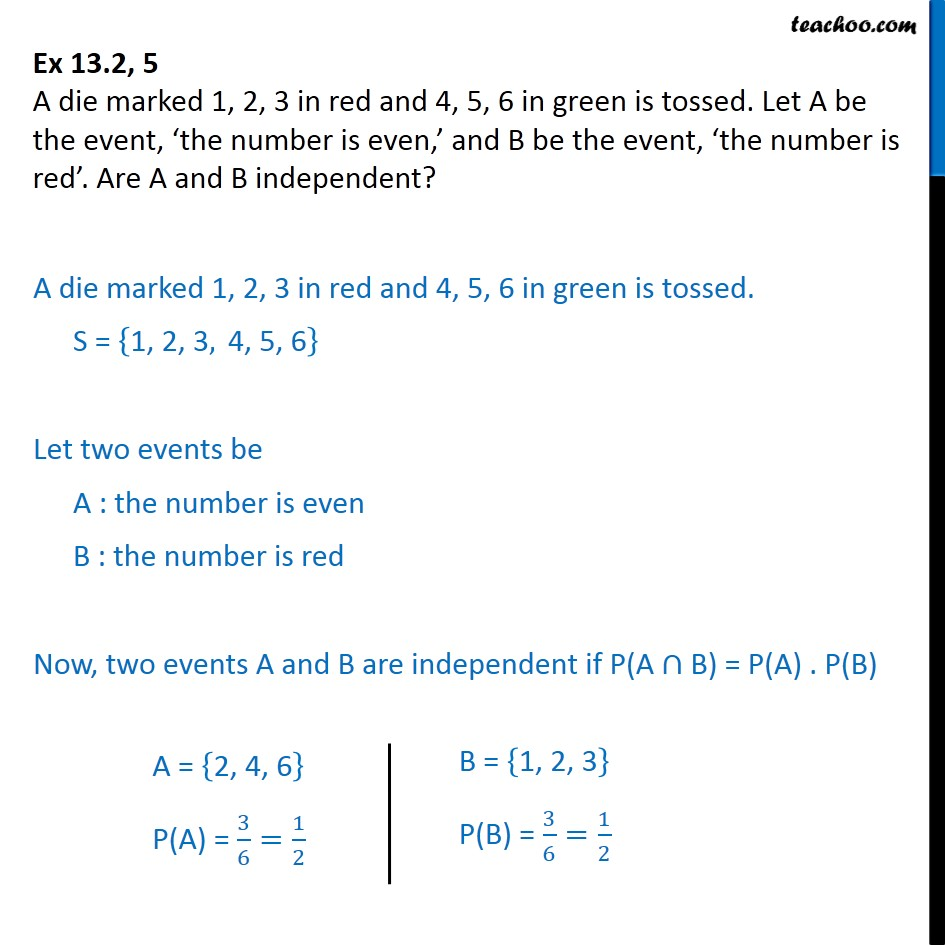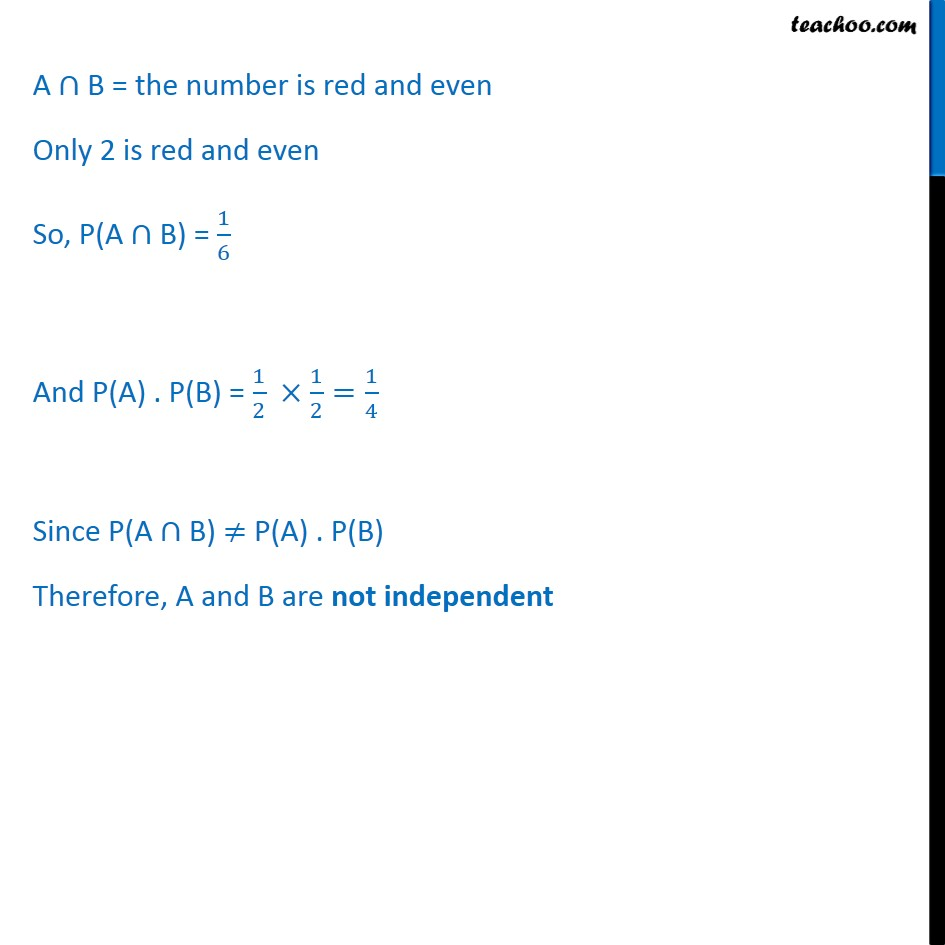Ex 13.2

Chapter 13 Class 12 Probability
Serial order wiseIntroducing your new favourite teacher - Teachoo Black, at only ₹83 per month

### Transcript

Ex 13.2, 5 A die marked 1, 2, 3 in red and 4, 5, 6 in green is tossed. Let A be the event, the number is even, and B be the event, the number is red . Are A and B independent? A die marked 1, 2, 3 in red and 4, 5, 6 in green is tossed. S = 1, 2, 3, 4, 5, 6 Let two events be A : the number is even B : the number is red Now, two events A and B are independent if P(A B) = P(A) . P(B) A B = the number is red and even Only 2 is red and even So, P(A B) = 1 6 And P(A) . P(B) = 1 2 1 2 = 1 4 Since P(A B) P(A) . P(B) Therefore, A and B are not independent